# 18 Results

View
Selected filters:
• Calculus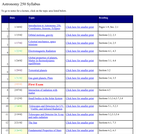Rating

This collection of lecture notes discusses the fundamentals of astronomy. The lecture notes review the topics of celestial mechanics, light, matter, planets, telescopes, the sun, stellar astronomy, stellar evolution, life in the universe, galaxies, and cosmology.

Subject:
Technology
Education
Mathematics
Calculus
Geometry
Physics
Space Science
Material Type:
Lecture Notes
Provider:
AMSER: Applied Math and Science Education Repository
Provider Set:
AMSER: Applied Math and Science Education Repository
ComPADRE: Resources for Physics and Astronomy Education
Author:
Marcia Rieke
Rieke, Marcia
07/09/2004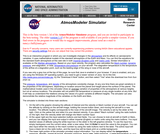Rating

Atmosphere Applet: This program lets you study how the properties of the atmosphere change with altitude. You can study the atmosphere of either the Earth or Mars. The equations used in this program are taken from the ICAO standard day model for the Earth and from some curve fits of the Martian atmosphere gathered by the Global Surveyor spacecraft. Using the airplane graphic you can select an altitude, or you can type an altitude into the input box.

The program instantly outputs a selected property and displays the local temperature and pressure on gauges You can output the temperature, pressure, density, local speed of sound, Mach number for specified velocity, or the ratio of aircraft lift to the lift on Earth at sea level. Input and output can be given in either English or metric units.

Subject:
Engineering
Algebra
Calculus
Functions
Trigonometry
Chemistry
Geoscience
Physics
Space Science
Material Type:
Full Course
Interactive
Provider:
NASA
Provider Set:
ComPADRE: Resources for Physics and Astronomy Education
NASA Glenn Research Center
Author:
NASA Glenn Research Center
Tom Benson
08/09/2008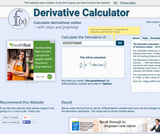Rating

An online derivative calculator that computes symbolic derivatives of mathematical expressions. User input and results are typeset as graphical formulas.

Subject:
Engineering
Mathematics
Calculus
Provider:
NSDL Staff
Provider Set:
Mathematics Gateways and Resources
Author:
David Scherfgen
11/04/2013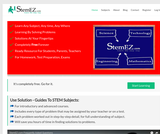Rating

Encyclopedia of STEM education is a free resource with 50,000 problems/solutions covering 13 math subjects, 13 science subjects and 13 engineering subjects for self study learning, classroom teaching, tutoring and teacher training.

Subject:
Engineering
Technology
Education
Mathematics
Algebra
Calculus
Functions
Geometry
Statistics and Probability
Trigonometry
Physical Science
Chemistry
Physics
Material Type:
Full Course
Homework/Assignment
Student Guide
Teaching/Learning Strategy
Author:
open source
12/21/2017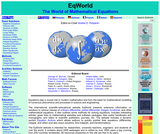Rating

This site provides information on solutions to various classes of mathematical equations. There are exact solutions and methods for solving ordinary differential equations, partial differential equations, integral equations, and other classes of functions and transforms. The Equation Archive allows authors to post equations and exact solutions, first integrals, and transformations. There is also an index of named algebraic, ordinary differential, partial differential, integral, and functional equations. Other materials include links to online forums, information on mathematical software, and links to educational materials, publications, and related websites.

Subject:
Mathematics
Algebra
Calculus
Provider:
NSDL Staff
Provider Set:
Mathematics Gateways and Resources
Author:
Andrei D. Polyanin
02/03/2006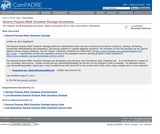Rating

The General Purpose Math Visualizer Package performs mathematical tasks that are commonly encountered in physics: plotting, animating, numerically differentiating and integrating, and solving systems of coupled algebraic equations.&nbsp;For examples of how this package can be used to explore various physics problems, see the chapter "Using the Computer as a Black Box" at http://www.compadre.org/osp/items/detail.cfm?ID=11330. A significantly revised and expanded version of this package’s function plotting program is also available at http://www.compadre.org/osp/items/detail.cfm?ID=11593. The General Purpose Math Visualizer Package was developed using the Easy Java Simulations (Ejs) modeling tool.&nbsp;&nbsp; It is distributed as a ready-to-run (compiled) Java archive. Double clicking the ejs_GeneralPurposeMath.jar file will run the program if Java is installed. An alternate version (ejs_GeneralPurposeMath_lowres.jar) is also included below that should fit on the screen more nicely when using a display set to a low resolution.

Subject:
Mathematics
Algebra
Calculus
Physics
Material Type:
Interactive
Provider:
Provider Set:
ComPADRE: Resources for Physics and Astronomy Education
Author:
Larry Engelhardt
06/18/2011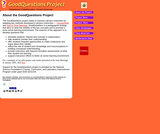Rating

GoodQuestions is a pedagogical strategy designed to improve calculus instruction for higher-level students by adapting two methods developed in physics instruction: ConcepTests (conceptual multiple-choice questions) and Just-in-Time Teaching (a pedagogical strategy that blends web technology with active learning in classroom situations). The project web site includes class materials, links to math classes where the strategy is being employed, news articles, and information on the project team.

Subject:
Mathematics
Calculus
Material Type:
Teaching/Learning Strategy
Provider:
NSDL Staff
Provider Set:
Mathematics Gateways and Resources
04/03/2004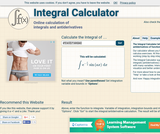Rating

An online integral calculator supporting definite integrals, indefinite integrals (antiderivatives) and improper integrals. User input and results are typeset as graphical formulas.

Subject:
Engineering
Mathematics
Calculus
Provider:
NSDL Staff
Provider Set:
Mathematics Gateways and Resources
Author:
David Scherfgen
11/04/2013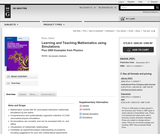Rating

This work takes an experimental approach to understanding mathematics through the use of interactive Java simulations. Suggestions for experiments to perform are included for over 60 interactive EJS models created by the author and a collection of over 2000 java simulations in physics. Topics covered include infinitesimal calculus, partial differential equations, fractals and much more. Intervention and customization of the calculation programs is possible using the EJS (Easy Java Simulation) simulation technology. A module library also allows users to engage in further development. In the electronic version of the text, the examples can be accessed directly from the text and operated online or from an installed package. The interactive format is an ideal tool to help users visualize and understand mathematics and physics and their simulation techniques.

Subject:
Computing and Information
Mathematics
Calculus
Physics
Material Type:
Textbook
Provider:
Provider Set:
ComPADRE: Resources for Physics and Astronomy Education
Author:
Dieter Röß
04/07/2012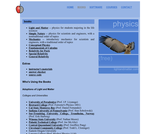Conditional Remix & Share Permitted
CC BY-SA
Rating

Light and Matter is a six-volume series of introductory physics textbooks.

This digital textbook was reviewed for its alignment with California content standards.

Subject:
Engineering
Education
Mathematics
Calculus
Physics
Material Type:
Diagram/Illustration
Lesson Plan
Textbook
Provider:
AMSER: Applied Math and Science Education Repository
Benjamin Crowell
NSDL Staff
Provider Set:
AMSER: Applied Math and Science Education Repository
Physics Gateways and Resources
Author:
Crowell, Benjamin
Dr. Benjamin Crowell
01/15/2007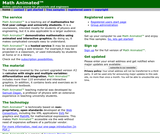Rating

This web site contains courseware for single and many-variables calculus designed for introductory undergraduate physics and engineering students. Included are text explanations and solved exercises, supported by animated and interactive graphics. The graphics make the material useful for a broader audience in both the classroom and by individual students. These materials use MathML and SVG. The free Firefox browser can be used to view, these resources without any plugins on Windows, Mac and Linux.

Subject:
Mathematics
Calculus
Physics
Material Type:
Lecture Notes
Provider:
Provider Set:
ComPADRE: Resources for Physics and Astronomy Education
Author:
Samuel Dagan
09/23/2005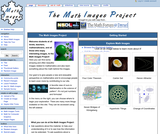Rating

This Wiki-based image gallery features imagery related to mathematics, with explanations of the math principles behind the images. Each image is accompanied by information on its creator, source, field of mathematics, a brief explanation of the principles behind the image, and links to additional information (where available). The gallery is searchable by keyword and browseable by title, author, field of mathematics, and grade level. Users are invited to contribute images and explanatory material to the gallery.

Subject:
Mathematics
Algebra
Calculus
Geometry
Geoscience
Material Type:
Diagram/Illustration
Provider:
NSDL Staff
Provider Set:
Mathematics Gateways and Resources
11/04/2014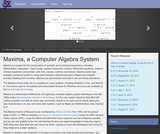Rating

Extracted from its web site: "Maxima is a system for the manipulation of symbolic and numerical expressions, including differentiation, integration, Taylor series, Laplace transforms, ordinary differential equations, systems of linear equations, polynomials, and sets, lists, vectors, matrices, and tensors. Maxima yields high precision numeric results by using exact fractions, arbitrary precision integers, and variable precision floating point numbers. Maxima can plot functions and data in two and three dimensions.... Maxima is a descendant of Macsyma, the legendary computer algebra system developed in the late 1960s at the Massachusetts Institute of Technology. It is the only system based on that effort still publicly available and with an active user community, thanks to its open source nature. Macsyma was revolutionary in its day, and many later systems, such as Maple and Mathematica, were inspired by it." Maxima is available free of charge under the GNU General Public License. There are versions for Linux, Windows, MaC OS X, as well as other operating systems. The Windows version installation includes the popular graphical environment wxMaxima which was specifically developed for it.

Subject:
Computing and Information
Mathematics
Algebra
Calculus
Physics
Material Type:
Data Set
Provider:
Provider Set:
ComPADRE: Resources for Physics and Astronomy Education
Author:
Dr. William Schelter
Maxima Users and Developers Group
sourceforge.net
11/21/2010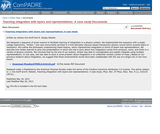Unrestricted Use
CC BY
Rating

We designed a sequence of seven lessons to facilitate learning of integration in a physics context. We implemented this sequence with a single college sophomore, “Amber,” who was concurrently enrolled in a first-semester calculus-based introductory physics course which covered topics in mechanics. We outline the philosophy underpinning these lessons, which characterizes integration in terms of layers and representations. We describe how Amber learned to give oral presentations in which she told a story about how integration comes from products, sums, and limits in a variety of physics contexts. We conclude that by the end of our lessons, Amber was able to conceptualize and explain integrals using multiple representations. In one case, she was able to solve a novel problem about integration in an unfamiliar context (center of mass.) Based on our previous research about integration, we suggest that these achievements would have been unattainable with the use of a single one or two hour lesson.

Subject:
Education
Mathematics
Calculus
Physics
Material Type:
Case Study
Provider:
Provider Set:
ComPADRE: Resources for Physics and Astronomy Education
Author:
Joshua Von Korff
N. Sanjay Rebello
05/20/2012Rating

In this calculus activity, learners use a classic problem of geometrical probability to find an important mathematical constant (pi). Learners explore Buffonäóťs needle problem (which asks the probability of a needle hitting a line if it is thrown at a group of evenly spaced parallel lines) by physically throwing toothpicks and graphing the results.

Subject:
Education
Mathematics
Calculus
Geometry
Material Type:
Activity/Lab
Provider:
Science and Math Informal Learning Educators (SMILE)
Provider Set:
SMILE Pathway: Science and Math Activities in One Search
Author:
Julie Yu
National Science Foundation
The Exploratorium
11/09/2009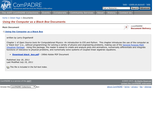Rating

Chapter 1 of Open Source tools for Computational Physics: An introduction to EJS and Python. This chapter introduces the use of the computer as a “black box” (i.e., without programming) for solving a variety of physics and engineering problems, making use of the General Purpose Math Visualizer Package. Using this package, the reader is asked to create and analyze plots and animations, numerically differentiate and integrate functions of relevance to physical problems, and numerically solve systems of coupled linear algebraic equations.

Subject:
Computing and Information
Education
Mathematics
Algebra
Calculus
Physics
Material Type:
Activity/Lab
Data Set
Interactive
Provider:
Provider Set:
ComPADRE: Resources for Physics and Astronomy Education
Author:
Larry Engelhardt
07/19/2011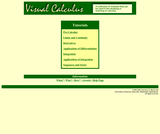Restricted Use
Rating

A collection of modules to be used in study or teaching of calculus which includes tutorials and detailed instructions for TI-85 and TI-86 graphing calculators.

Subject:
Computing and Information
Education
Mathematics
Calculus
Geoscience
Physics
Material Type:
Activity/Lab
Assessment
Diagram/Illustration
Interactive
Lecture Notes
Student Guide
Provider:
AMSER: Applied Math and Science Education Repository
Internet Scout Project
Science Education Resource Center (SERC) at Carleton College
Shodor
Provider Set:
AMSER: Applied Math and Science Education Repository
ComPADRE: Resources for Physics and Astronomy Education
Internet Scout Project
Starting Point: Teaching Entry Level Geoscience
CSERD: Computational Science Education Reference Desk
Author:
Alexa Bosse
Chris O'Brien
Eric Howell
Innovative Technology Center, University of Tennessee, Knoxville
Lawrence Husch
Lawrence S. Husch
Marek Szapiel
Richard Rupp
University of Tennessee, Knoxville
10/10/2003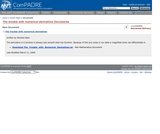Rating

The derivative of a function is always less smooth than the function. Because of this any noise in our data is magnified when we differentiate it.

Subject:
Computing and Information
Mathematics
Calculus
Physics
Material Type:
Activity/Lab
Unit of Study
Provider: